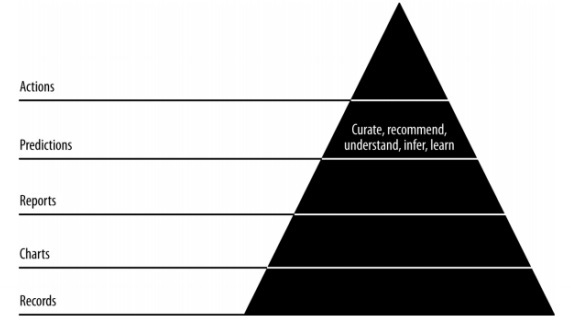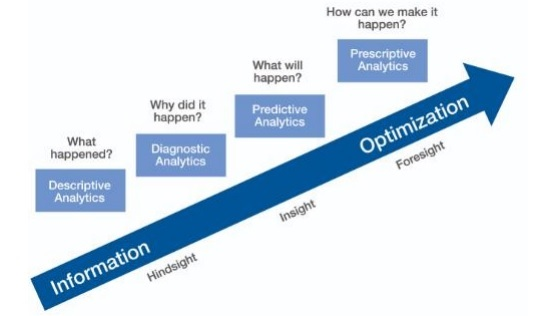# Agile Data Science - Role of Predictions

In this chapter, we will earn about the role of predictions in agile data science. The interactive reports expose different aspects of data. Predictions form the fourth layer of agile sprint.When making predictions, we always refer to the past data and use them as inferences for future iterations. In this complete process, we transition data from batch processing of historical data to real-time data about the future.

The role of predictions includes the following −

• Predictions help in forecasting. Some forecasts are based on statistical inference. Some of the predictions are based on opinions of pundits.

• Statistical inference are involved with predictions of all kinds.

• Sometimes forecasts are accurate, while sometimes forecasts are inaccurate.

## Predictive Analytics

Predictive analytics includes a variety of statistical techniques from predictive modeling, machine learning and data mining which analyze current and historical facts to make predictions about future and unknown events.

Predictive analytics requires training data. Trained data includes independent and dependent features. Dependent features are the values a user is trying to predict. Independent features are features describing the things we want to predict based on dependent features.

The study of features is called feature engineering; this is crucial to making predictions. Data visualization and exploratory data analysis are parts of feature engineering; these form the core of Agile data science.## Making Predictions

There are two ways of making predictions in agile data science −

• Regression

• Classification

Building a regression or a classification completely depends on business requirements and its analysis. Prediction of continuous variable leads to regression model and prediction of categorical variables leads to classification model.

### Regression

Regression considers examples that comprise features and thereby, produces a numeric output.

### Classification

Classification takes the input and produces a categorical classification.

Note − The example dataset that defines input to statistical prediction and that enables the machine to learn is called “training data”.